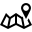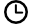# Κατάλογος Εκδηλώσεων

02
Ιουν

Ομιλία κ. Antonios Varvitsiotis " Non-commutative Extension of Lee-Seung's Algorithm for Positive Semidefinite Factorizations"
Κατηγορία: Ομιλία/ΔιάλεξηΗ ομιλία θα γίνει με τηλεδιάσκεψη02/06/2021 13:00 - 14:00

Περιγραφή:

Antonios Varvitsiotis, Assistant Professor at the Singapore University of Technology and Design

Title
A Non-commutative Extension of Lee-Seung's Algorithm for Positive Semidefinite Factorizations

Abstract
Given a data matrix $X\in \mathbb{R}_+^{m\times n}$ with non-negative entries, a Positive Semidefinite (PSD) factorization of $X$ is a collection of $r \times r$-dimensional PSD matrices $\{A_i\}$ and $\{B_j\}$ satisfying the condition $X_{ij}= \mathrm{tr}(A_i B_j)$ for all $\ i\in [m],\ j\in [n]$.  PSD factorizations are fundamentally linked to understanding the expressiveness of semidefinite programs as well as the power and limitations of quantum resources in information theory.  The PSD factorization task generalizes the Non-negative Matrix Factorization (NMF) problem in which we seek a collection of $r$-dimensional non-negative vectors $\{a_i\}$ and $\{b_j\}$ satisfying $X_{ij}= a_i^T b_j$,  for all $i\in [m],\ j\in [n]$ -- one can recover the latter problem by choosing matrices in the PSD factorization to be diagonal.  The most widely used algorithm for computing NMFs of a matrix is the Multiplicative Update algorithm developed by Lee and Seung, in which non-negativity of the updates is preserved by scaling with positive diagonal matrices.  In this paper, we describe a non-commutative extension of Lee-Seung's algorithm, which we call the Matrix Multiplicative Update (MMU) algorithm, for computing PSD factorizations.  The MMU algorithm ensures that updates remain PSD by congruence scaling with the matrix geometric mean of appropriate PSD matrices, and it retains the simplicity of implementation that the multiplicative update algorithm for NMF enjoys.  Building on the Majorization-Minimization framework, we show that under our update scheme the squared loss objective is non-increasing and fixed points correspond to critical points.  The analysis relies on  Lieb's Concavity Theorem.  Beyond PSD factorizations, we show that the MMU algorithm can be also used as a primitive to calculate block-diagonal PSD factorizations and tensor PSD factorizations.  We demonstrate the utility of our method with experiments on real and synthetic data.

Joint work with Yong Sheng Soh, National University of Singapore.

About the speaker
https://sites.google.com/site/antoniosvarvitsiotis/

Assistant Professor Varvitsiotis received a PhD in Mathematical Optimization from the Dutch National Research Institute for Mathematics and Computer Science (CWI). Prior to joining the Singapore University of Technology and Design he held Research Fellow positions at the Centre for Quantum Technologies (Computer Science group) and the National University of Singapore (Department of Electrical and Computer Engineering and Department of Industrial and Systems Engineering). He also holds a MSc degree in Theoretical Computer Science and a BSc in Applied and Theoretical Mathematics, both from the National University of Athens in Greece. Dr. Varvitsiotis’ research is focused on fundamental aspects of continuous and discrete optimisation, motivated by real-life applications in data science, engineering, and quantum information.

Meeting ID: 923 5451 8920
Password: 953377

© Σχολή Ηλεκτρολόγων Μηχανικών & Μηχανικών Υπολογιστών 2014
Πολυτεχνείο Κρήτης